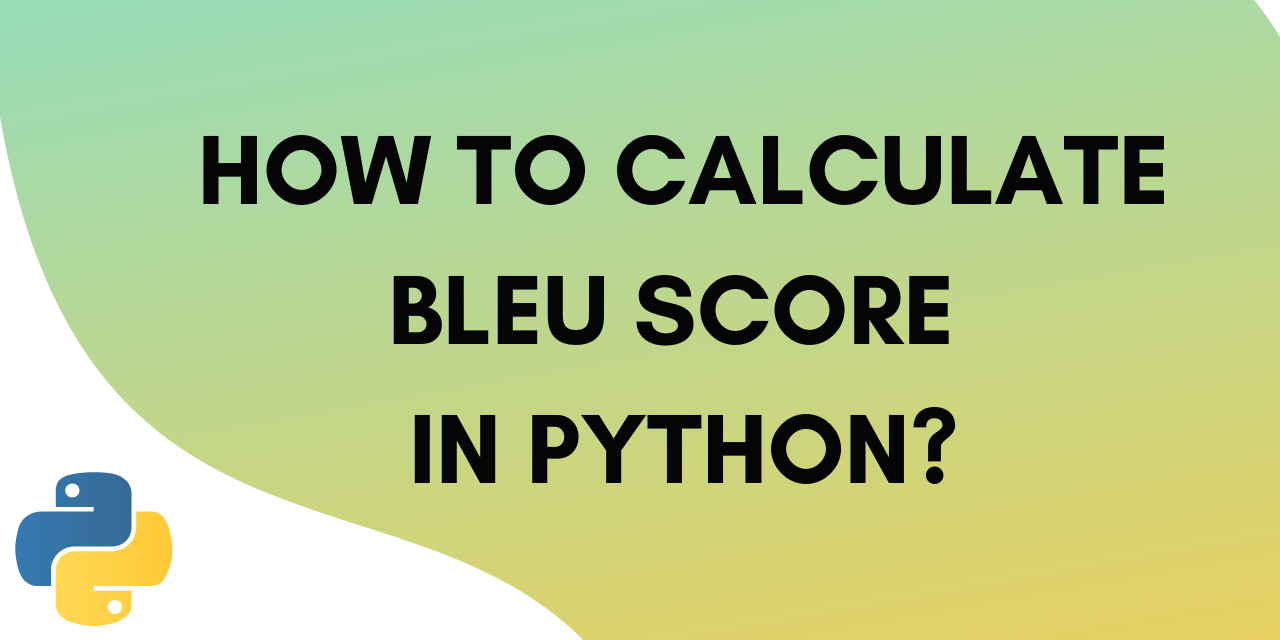# How to calculate BLEU Score in Python?

Published on August 3, 2022By Jayant VermaWhile we believe that this content benefits our community, we have not yet thoroughly reviewed it. If you have any suggestions for improvements, please let us know by clicking the “report an issue“ button at the bottom of the tutorial.

Bleu score in Python is a metric that measures the goodness of Machine Translation models. Though originally it was designed for only translation models, now it is used for other natural language processing applications as well.

The BLEU score compares a sentence against one or more reference sentences and tells how well does the candidate sentence matched the list of reference sentences. It gives an output score between 0 and 1.

A BLEU score of 1 means that the candidate sentence perfectly matches one of the reference sentences.

This score is a common metric of measurement for Image captioning models.

In this tutorial, we will be using  sentence_bleu() function from the nltk library. Let’s get started.

## Calculating the Bleu score in Python

To calculate the Bleu score, we need to provide the reference and candidate sentences in the form of tokens.

We will learn how to do that and compute the score in this section. Let’s start with importing the necessary modules.

``````from nltk.translate.bleu_score import sentence_bleu
``````

Now we can input the reference sentences in the form of a list. We also need to create tokens out of sentences before passing them to the sentence_bleu() function.

### 1. Input and Split the sentences

The sentences in our reference list are:

``````    'this is a dog'
'it is dog
'dog it is'
'a dog, it is'
``````

We can split them into tokens using the split function.

``````reference = [
'this is a dog'.split(),
'it is dog'.split(),
'dog it is'.split(),
'a dog, it is'.split()
]
print(reference)
``````

Output :

``````[['this', 'is', 'a', 'dog'], ['it', 'is', 'dog'], ['dog', 'it', 'is'], ['a', 'dog,', 'it', 'is']]
``````

This is what the sentences look like in the form of tokens. Now we can call the sentence_bleu() function to calculate the score.

### 2. Calculate the BLEU score in Python

To calculate the score use the following lines of code:

``````candidate = 'it is dog'.split()
print('BLEU score -> {}'.format(sentence_bleu(reference, candidate)))
``````

Output :

``````BLEU score -> 1.0
``````

We get a perfect score of 1 as the candidate sentence belongs to the reference set. Let’s try another one.

``````candidate = 'it is a dog'.split()
print('BLEU score -> {}'.format(sentence_bleu(reference, candidate)))
``````

Output :

``````BLEU score -> 0.8408964152537145
``````

We have the sentence in our reference set, but it isn’t an exact match. This is why we get a 0.84 score.

### 3. Complete Code for Implementing BLEU Score in Python

Here’s the complete code from this section.

``````from nltk.translate.bleu_score import sentence_bleu
reference = [
'this is a dog'.split(),
'it is dog'.split(),
'dog it is'.split(),
'a dog, it is'.split()
]
candidate = 'it is dog'.split()
print('BLEU score -> {}'.format(sentence_bleu(reference, candidate )))

candidate = 'it is a dog'.split()
print('BLEU score -> {}'.format(sentence_bleu(reference, candidate)))
``````

### 4. Calculating the n-gram score

While matching sentences you can choose the number of words you want the model to match at once. For example, you can choose for words to be matched one at a time (1-gram). Alternatively, you can also choose to match words in pairs (2-gram) or triplets (3-grams).

In this section we will learn how to calculate these n-gram scores.

In the sentence_bleu() function you can pass an argument with weights corresponding to the individual grams.

For example, to calculate gram scores individually you can use the following weights.

``````Individual 1-gram: (1, 0, 0, 0)
Individual 2-gram: (0, 1, 0, 0).
Individual 3-gram: (1, 0, 1, 0).
Individual 4-gram: (0, 0, 0, 1).
``````

Python code for the same is given below:

``````from nltk.translate.bleu_score import sentence_bleu
reference = [
'this is a dog'.split(),
'it is dog'.split(),
'dog it is'.split(),
'a dog, it is'.split()
]
candidate = 'it is a dog'.split()

print('Individual 1-gram: %f' % sentence_bleu(reference, candidate, weights=(1, 0, 0, 0)))
print('Individual 2-gram: %f' % sentence_bleu(reference, candidate, weights=(0, 1, 0, 0)))
print('Individual 3-gram: %f' % sentence_bleu(reference, candidate, weights=(0, 0, 1, 0)))
print('Individual 4-gram: %f' % sentence_bleu(reference, candidate, weights=(0, 0, 0, 1)))

``````

Output :

``````Individual 1-gram: 1.000000
Individual 2-gram: 1.000000
Individual 3-gram: 0.500000
Individual 4-gram: 1.000000
``````

Be default the sentence_bleu() function calculates the cumulative 4-gram BLEU score, also called BLEU-4. The weights for BLEU-4 are as follows :

``````(0.25, 0.25, 0.25, 0.25)
``````

Let’s see the BLEU-4 code:

``````score = sentence_bleu(reference, candidate, weights=(0.25, 0.25, 0.25, 0.25))
print(score)
``````

Output :

``````0.8408964152537145
``````

That’s the exact score we got without the n-gram weights added.

## Conclusion

This tutorial was about calculating the BLEU score in Python. We learned what it is and how to calculate individual and cumulative n-gram Bleu scores. Hope you had fun learning with us!

Thanks for learning with the DigitalOcean Community. Check out our offerings for compute, storage, networking, and managed databases.Jayant Verma

author

#### Still looking for an answer?

Ask a questionSearch for more help

Click below to sign up and get \$200 of credit to try our products over 60 days!

### Popular Topics##### Hollie's Hub for Good

Working on improving health and education, reducing inequality, and spurring economic growth? We’d like to help.##### Become a contributor

You get paid; we donate to tech nonprofits.

##### Welcome to the developer cloud

DigitalOcean makes it simple to launch in the cloud and scale up as you grow – whether you’re running one virtual machine or ten thousand.© 2023 DigitalOcean, LLC.